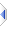# power

 Definition: atom x3 = power(atom x1, atom x2) Description: Raise x1 to the power x2 Comments: The arguments to this function must be atoms. Use sq_power() if either or both parameters might be sequences. The rules for sequence operations apply. Powers of 2 are calculated very efficiently. Other languages have a ** or ^ operator to perform the same action, although in some languages ^ is the xor function. Theoretically the result of power(0,0) is undefined, however for consistency with other programming languages, the result is 1. However, in phix (the same as python), attempting to raise any value <=0 to any negative or non integer power causes a fatal runtime error. Obvious workarounds exist, for instance should you require a function that returns the cubic root of negative (and positive) numbers: ```function cube_root(atom c) return iff(c>=0?power(c,1/3):-power(-c,1/3)) -- return iff(c>=0?1:-1)*power(abs(c),1/3)) -- (also fine) -- return compare(c,0)*power(abs(c),1/3) -- (ditto) end function ``` Example 1: ```? power(5, 2) -- 25 is printed ``` Example 2: ```? sq_power({5, 4, 3.5}, {2, 1, -0.5}) -- {25, 4, 0.534522} is printed ``` Example 3: ```? sq_power(2, {1, 2, 3, 4}) -- {2, 4, 8, 16} is printed ``` Example 4: ```? sq_power({1, 2, 3, 4}, 2) -- {1, 4, 9, 16} is printed ``` Implementation: via :%opPow in builtins\VM\pPower.e (an autoinclude) See Also: log, sqrt, iff